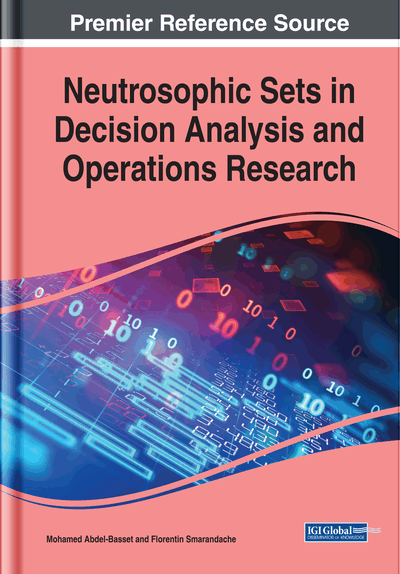# A Novel Python Toolbox for Single and Interval-Valued Neutrosophic Matrices

Said Broumi (Laboratory of Information Processing, Faculty of Science Ben M'Sik, University Hassan II, Morocco), Selçuk Topal (Faculty of Science and Arts, Bitlis Eren University, Turkey), Assia Bakali (Ecole Royale Navale, Casablanca, Morocco), Mohamed Talea (Laboratory of Information Processing, Faculty of Science Ben M'Sik, University Hassan II, Morocco) and Florentin Smarandache (Department of Mathematics, University of New Mexico, USA)
DOI: 10.4018/978-1-7998-2555-5.ch013
Available
\$37.50
No Current Special Offers

## Abstract

Recently, single valued neutrosophic sets and interval valued neutrosophic sets have received great attention among the scholars and have been applied in many applications. These two concepts handle the indeterminacy and consistent information existing in real-life problems. In this chapter, a new Python toolbox is proposed under neutrosophic environment, which consists of some Python code for single valued neutrosophic matrices and interval valued neutrosophic matrices. Some definitions of interval neutrosophic vague set such as union, complement, and intersection are presented. Furthermore, the related examples are included.
Chapter Preview
Top

## 1. Introduction

The introduction of the idea of fuzzy set was introduced by (Zadeh, 1965). He proposed that each element in a fuzzy set has a degree of membership. Based on this idea Dr (Atanassov,1986) introduced the concept of intuitionistic fuzzy set on a universe X as a generalization of fuzzy set. His concept is based on two degrees for each element: the degree of membership and degree of non-membership. Later on, Dr (Smarandache,1998) originally gave the definition of a neutrosophic set and neutrosophic logic. The concept of neutrosophic set is based on three degrees for each element totally independent: the degree of membership the degree of indtermination and degree of non-membership. In (Wang et al, 2014) proposed the concept of single valued neytrosophic set which is an extensin of Neutrosophic set. In addition, the author (Zhang et al.,2014) developped a new extension termed as interval valued neutrosophic set.Several scholars have done remarkable achievements in this area (http://fs.gallup.unm.edu/NSS/).

(Biswas et al, 2018)applied the concept of interval trapezoidal neutrosophic numbers for solving MADM Strategy based distance. (Giri et al, 2018) developed TOPSIS Method for MADM based on interval trapezoidal neutrosophic number. (Hazwani et al,2019) introduced the concept of interval neutrosophic vague sets,this concept is acombination of interval valued neutrosophic set and vague sets, the author presented some definitions of interval neutrosophic vague set such as union, complement and intersection with including the basic operations, the derivation of its properties and related examples. (Nagarajan et al., 2019) proposed blockchain single and interval valued neutrosophic graphs and sutdied some of their proprtites with numerical examples.In (Broumi et al., 2019) applied the concept of interval valued neutrosophic graph for solving energy and spectrum analysis using MATLAB software. (Nagarajan et al.,2019) initiated the Dombi interval valued neutrosophic graph and its role in traffic control management. (Kalyan Mondal etal.,2018) applied the interval neutrosophic tangent similarity measure for solving MADM strategy. In (Pramanik et al,2018) applied the concept of interval rough neutrosophic sets for solving multi attribute decision making strategy based on projection and bidirectional projection measures. (Song et al.,2017) applied the interval neutrosophic sets applied to ideals in BCK/BCI-algebras. (Dalapatiet al.,2017) defined the interval neutrosophic -cross entropy for solving MAGDM Strategy. (Dalbinder and Kajla,2017) solved the selection of transportation companies and their mode of transportation under interval valued data. (Mukherjee et al.,2016) defined the restricted interval valued neutrosophic sets and restricted interval valued neutrosophic topological spaces.

## Key Terms in this Chapter

Toolbox: Programs or functions accessible from a single menu.

Python: A programming language which is useful for scientific computations and implementations.

Single Valued Neutrosophic Set: Set of neutrosophic elements dependent to a variable.

Matrix Operations: Operations that are defined on matrices.

Interval Valued Neutrosophic Set: Neutrosophic set of element components are intervals.

Implementation: A realization of a technical specification or algorithm as a program.

## Complete Chapter List

Search this Book:
Reset